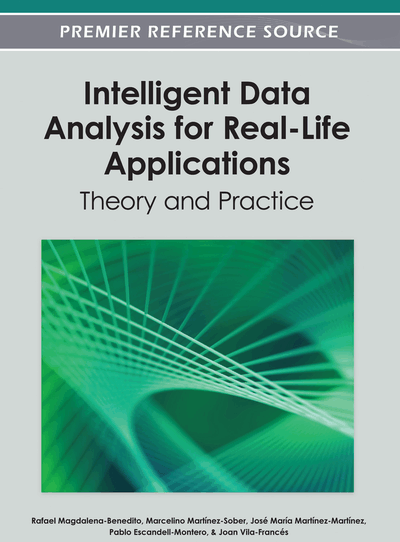# Supervised Classification with Bayesian Networks: A Review on Models and Applications

M. Julia Flores (University of Castilla – La Mancha (UCLM), Instituto de Investigación en informática de Albacete, Spain), José A. Gámez (University of Castilla – La Mancha (UCLM), Instituto de Investigación en informática de Albacete, Spain) and Ana M. Martínez (University of Castilla – La Mancha (UCLM), Instituto de Investigación en informática de Albacete, Spain)
DOI: 10.4018/978-1-4666-1806-0.ch005
Available
\$37.50
No Current Special Offers

## Abstract

Bayesian Network classifiers (BNCs) are Bayesian Network (BN) models specifically tailored for classification tasks. There is a wide range of existing models that vary in complexity and efficiency. All of them have in common the ability to deal with uncertainty in a very natural way, at the same time providing a descriptive environment. In this chapter, the authors focus on the family of semi-naïve Bayesian classifiers (naïve Bayes, AODE, TAN, kDB, etc.), motivated by the good trade-off between efficiency and performance they provide. The domain of the BNs is generally of discrete nature, but since the presence of continuous variables is very common, the chapter discusses more classical and novel approaches to handling numeric data. In this chapter the authors also discuss more recent techniques such as multi-dimensional and dynamic models. Last but not least, they focus on applications and recent developments, including some of the BNCs approaches to the multi-class problem together with other traditionally successful and cutting edge cases regarding real-world applications.
Chapter Preview
Top

## Introduction

The task of classification is one of the most popular and, therefore, important tasks in data mining, as it is applied in many real applications. In this context, the basic classification task involves learning a model (or generalization) from a set of labelled data, in order to assign one label to every new example. The model learning phase can be more or less complex, to such a degree that most of the work might be carried out in the assignation phase (as in lazy classifiers) or, often simply called, classification phase. As world is not deterministic, we will have to manage with uncertainty in classification.

Formally, a model is learnt from a dataset with t examples and n attributes, all of them with known labels given by a special attribute called class, C. Hence it is also often referred to as supervised classification in contrast to unsupervised classification or clustering, where the labels are not known a priori. For every example of the typewhere each ai is the value for the attribute Ai, a typical classifier would assign a label ci from a finite setof possible labels. Note that we are referring here to a finite set of labels, if the class to predict is of a continuous type it becomes a problem of regression, which is out of the scope of this chapter.

There exist multiple paradigms for classification, such as Bayesian networks, decision trees, rule induction, artificial neural networks, genetic programming, support vector machines, etc. In this chapter we focus on the first of these, which might be seen as a combination of statistical techniques and graphical models. BNs provide several advantages to the classification task:

• The networks store information about dependencies existing among the variables involved, which makes them capable of inherently dealing with uncertainty, very frequently present in real world.

• The graphical representation through the Bayesian network facilitates the interpretation and formulation of conclusions about the domain of study.

• In addition, Bayesian network classifiers can combine causal relationships with probabilistic logic, which helps to incorporate expert knowledge into the model.

Thus, one of the greatest advantages of the BNs is that they can represent both the qualitative and the quantitative aspect of the problem. The former is encoded in a directed acyclic graph (DAG), whereas the latter involves storing a probability table for every node conditioned on its parents. Even though the conditional probability distribution can be represented in several ways, the most common representation is the use of tables, i.e. conditional probability tables (CPT).

In a DAG, each node represents a variable; an arc represents a direct dependence between the pair of nodes connected. If there is a directed arc from X to Y, it means that X is the parent of Y and Y is child of X. Furthermore, if there exists a directed path from X to Z, it implies that X is an ancestor of Z, while Z is a descendant of X.

Through the property of conditional independence, which states that a node is conditionally independent of its non-descendant given its parents, we can represent the joint probability distribution of a Bayesian network by the product of the CPTs associated with each of its nodes.

In classification, we want to obtaini.e. the conditional probability for C givenThe accurate estimation of the probabilities a posteriori for every combination of the class labels and the values of the attributes is unfeasible in practice, as it requires a large amount of training data even with a moderate number of attributes. That is why it is convenient to resort to the Bayes theorem:

## Complete Chapter List

Search this Book:
Reset